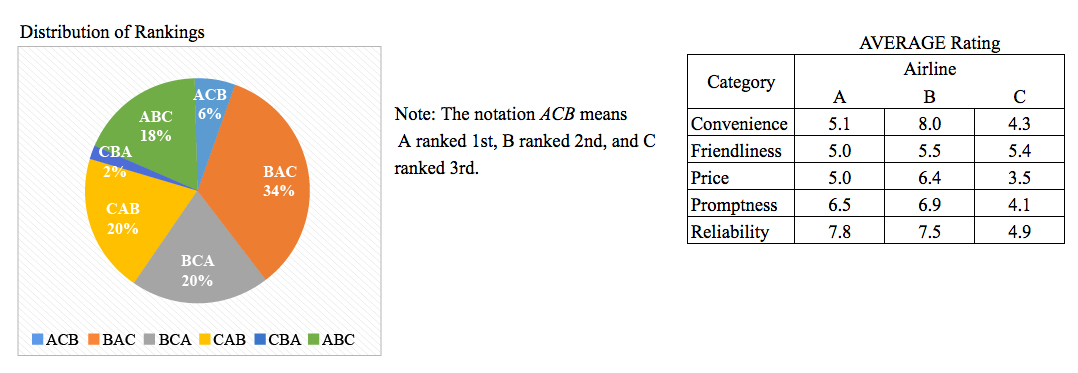# If each of the average ratings was the arithmetic mean of the

In a survey, \$100\$ travel agents each ranked Airlines \$A\$, \$B\$, and \$C\$ in order of preference. Each of the \$100\$ travel agents also rated the three airlines in five categories on a scale of \$1\$ through \$10\$, with \$10\$ being the best rating.If each of the average ratings was the arithmetic mean of the ratings given by the \$100\$ travel agents, approximately how much greater was the total of the ratings given to all three airlines for reliability than that for promptness?

1. \$25\$
2. \$50\$
3. \$125\$
4. \$250\$
5. \$500\$

So, you were trying to be a good test taker and practice for the GRE with PowerPrep online. Buuuut then you had some questions about the quant section—specifically question 16 of Section 4 of Practice Test 1. Those questions testing our knowledge of Graphical Methods for Describing Data can be kind of tricky, but never fear, PrepScholar has got your back!

## Survey the Question

Let’s search the problem for clues as to what it will be testing, as this will help shift our minds to think about what type of math knowledge we’ll use to solve this question. Pay attention to any words that sound math-specific and anything special about what the numbers look like, and mark them on our paper.

We have both a pie graph and a table containing numerical data, so this question likely tests what we know about Graphical Methods for Describing Data. Let’s keep what we’ve learned about this skill at the tip of our minds as we approach this question.

## What Do We Know?

Let’s carefully read through the question and make a list of the things that we know.

1. We want to calculate the difference between the total reliability rating for all three airlines combined and the total promptness rating for all three airlines combined
2. The convenience ratings are provided in a table

## Develop a Plan

The table gives us average values, but the question is looking for a total value, or sum. Thinking of an equation relating the two, we remember that:

\$\$\Average = {\Sum \of \Values}/{\Number \of \Values}\$\$

Since the question is looking for the sum of the values, let’s solve for this variable:

\$\$\Sum \of \Values = \Average·\Number \of \Values\$\$

Since there are \$100\$ travel agents providing the ratings, the number of values is equal to \$100\$. The “Average” that we are looking for is the average difference between reliability and promptness, added together for all three airlines. So let’s subtract promptness from reliability for each airline, add these values together, then multiply by \$100\$ to convert this average into a sum.

## Solve the Question

We know that the combined difference between the promptness and reliability ratings can be found by adding together these differences between each of the three airlines.

\$\$\Average \Difference = (A_{\Reliability}-A_{\Promptness})+(B_{\Reliability}-B_{\Promptness})+(C_{\Reliability}-C_{\Promptness})\$\$

We can get these six promptness and reliability averages for Airlines \$A\$, \$B\$, and \$C\$ from the table and simplify the right side of the equation.

 \$\Average \Difference\$ \$=\$ \$(7.8-6.5)+(7.5-6.9)+(4.9-4.1)\$ \$ \$ \$ \$ \$\Average \Difference\$ \$=\$ \$1.3+0.6+0.8\$ \$ \$ \$ \$ \$\Average \Difference\$ \$=\$ \$2.7\$

So for each travel agent, the sum of the average differences between the promptness and reliability ratings for all three airlines combined was \$2.7\$. We can get the sum of the average differences by multiplying by the number of travel agents. Doing so, we get \$2.7·100=270\$. Looking for the answer choice closest to \$270\$, we can see that the correct answer is D, \$250\$.

## What Did We Learn

Though the concept tested in this question wasn’t too difficult by GRE standards, it was definitely worded in a confusing way, where we needed to find the sum of the average of the difference of averages (say that three times fast!). We should always slow down and read these types of questions deliberately to minimize the likelihood of incorrectly assessing what the question is asking for.

Want more expert GRE prep? Sign up for the five-day free trial of our PrepScholar GRE Online Prep Program to access your personalized study plan with 90 interactive lessons and over 1600 GRE questions.